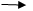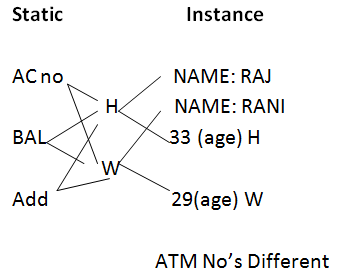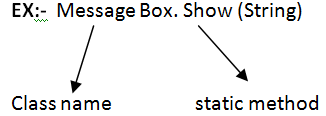# Static Keyword in C# .Net

14 October, 2020

Ratings

## Working with Static Keyword

Class Circle { Private int x, y, z; Private Static double pi = 3.14;. } Static is a keyword. Static keyword can be used with fields, constructors, methods, and classes. Static Fields will be created only once while the class is loaded into the memory. Instance Variables will be created separately with every object. Static variables create common memory which is sharable by all the objects. If the Static variable is public, then it can be accessed directly with the class name But NOT WITH OBJECTS.

Observations

``````Class Test

{

Public int I;

Public Static int S;

}

Test I = 10;

wrong Test s =20;

correct Test t = new Test ()

I = 10; correct

t.s = 20;

wrong

``````

A static constructor can access only static variables.

Static constructor will be executed only once, While class is loading into the memory.

Example of static variables and static constructor

Open windows form Application ProjectPlace a buttonCode in GD

``````Class Test

{

Private int I;

Private Static int s;

Static Test ()

// Static constructor

{

S= 0;

}

Public Test ()

//Normal Constructor

{

I = I +1;

S = s+1;

Message Box>show  (I + “” +s);

}

}

//Test   Code for button 1_ click

{

Test t1 = new Test ();

Test t2 = new Test ();

Test t3 = new Test ();

}

``````

S=0

T1  I =0

I=0 +1

I =1

S=1

I      s

1         1

T2   I =0

I = 0+1

I =1

S =s +1

S =I +1

S= 2

I     s

I     2        t2

I   s

I     3                  t3

### Observations

Class JA  (Joint account) {

Private string Name ()## Static Method and Static Classes

Class Test

{

Public Static void P1 ()

{}

Public Static void P2 ()

{}

}

A static method can access only static data. The static method needs to be called with the class name.When a class contains all static methods only. Then recommended to declare that class as static. Static class are not intangible (creation of an object is not allowed)

Example of static method and static classes

Open windows Application Project àPlace a text box and a button

### NoteIn this program, a predefined class called a process is required.Process class is a  part of the System. Diagnostics namespace.In order to use process classes, this namespace needs to be imported with the help of the “using”  keyword.Code in GD Static classes software { Public static void open (string s) { Process. start (s); }//open }//software classcode for Button 1-cilck { Software. open (text Box1.text); }